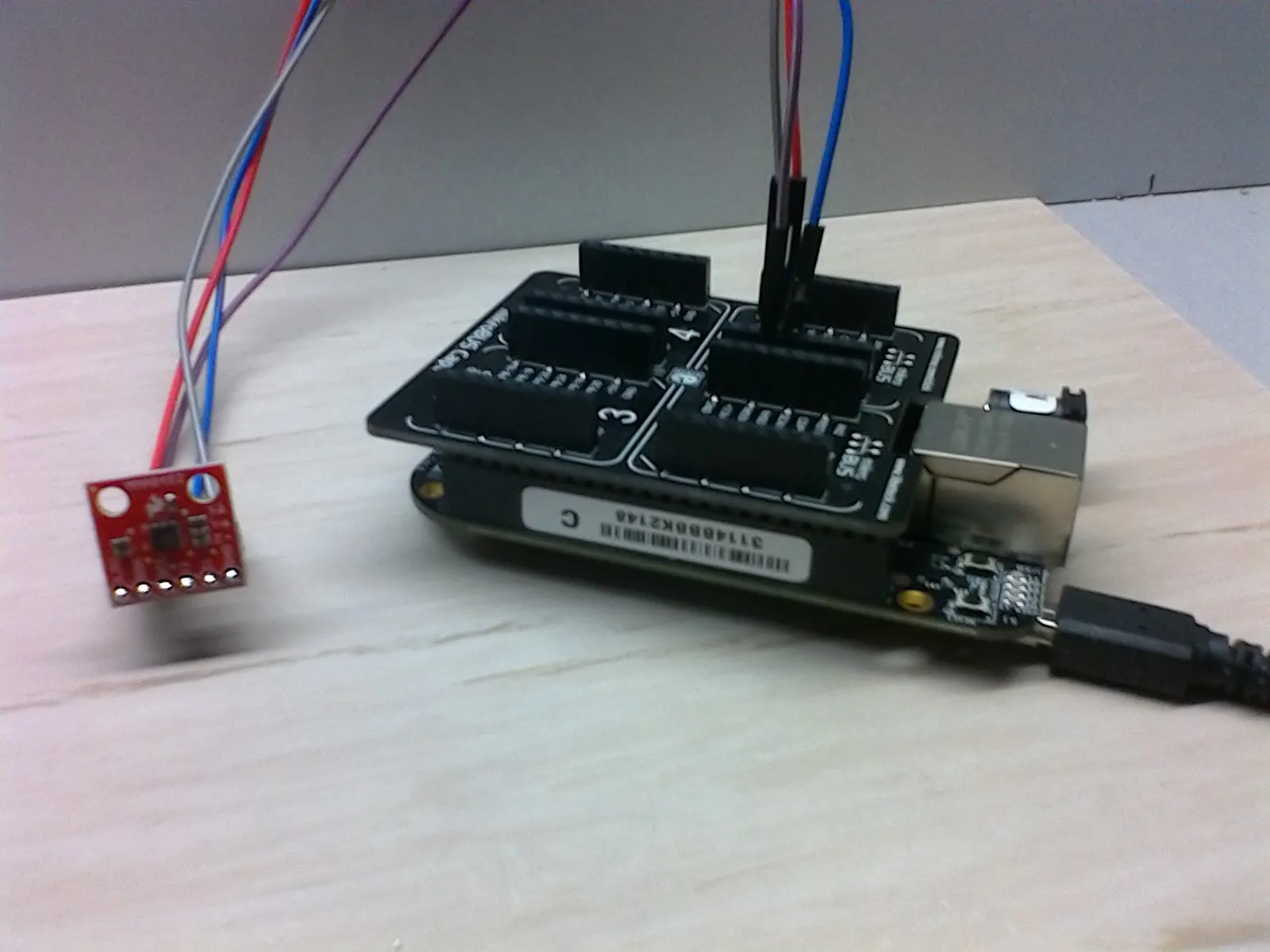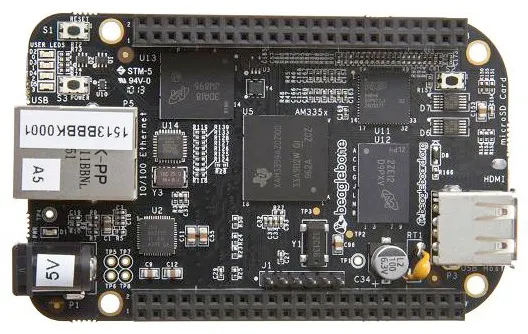# BeagleBone with Accelerometer MM8452

Simple article for BeagleBone and Sparkfun MM8452 accelerometer. Python code is used.

BeginnerProtip30 minutes1,652## Things used in this project

### Hardware componentsBeagleBoard.org BeagleBone Black
×1
 MikroElektronika MicroBUS Cape
×1
 SparkFun Triple Axis Accelerometer MMA8452Q
×1

## Code

### mm8452.py

Python
Python library for MMA8452 breakout board
```from Adafruit_I2C import Adafruit_I2C

def twos_comp(val, bits):
"""compute the 2's compliment of int value val"""
if (val & (1 << (bits - 1))) != 0: # if sign bit is set e.g., 8bit: 128-255
val = val - (1 << bits)        # compute negative value
return val

class MM8452_ACCEL :
i2C = None
scale = None
SCALE_2G = 2
SCALE_4G = 4
SCALE_8G = 8
ODR_800 = 0 # output data rate
ODR_400 = 1
ODR_200 = 2
ODR_100 = 3
ODR_50 = 4
ODR_12 = 5
ODR_6 = 6
ODR_1 = 7
WHO_AM_I = 0x0D
STATUS = 0x00
OUT_X_MSB = 0x01
OUT_X_LSB = 0x02
OUT_Y_MSB = 0x03
OUT_Y_LSB = 0x04
OUT_Z_MSB = 0x05
OUT_Z_LSB = 0x06
CTRL_REG1 = 0x2A
XYZ_DATA_CFG = 0x0E

def whoAmI(self):
return reg_val

def standby(self):
reg_val = reg_val & 0xFE # clear the active bit
self.i2C.write8(self.CTRL_REG1,reg_val)

def active(self):
reg_val = reg_val | 0x01 # set the active bit
self.i2C.write8(self.CTRL_REG1,reg_val)

def config(self, fsr = 2, odr = 0):
self.scale = fsr
self.standby()
self.setScale(self.scale)
self.setODR(odr)
self.active()

def setScale(self, scale):
# must be in standby mode!
reg_val = reg_val & 0xFC
scale = scale >> 2
reg_val = reg_val | scale
self.i2C.write8(self.XYZ_DATA_CFG,reg_val)

def setODR(self,odr):
reg_val = reg_val & 0xCF # clear the active bit
odr = odr << 3
reg_val = reg_val | odr
self.i2C.write8(self.CTRL_REG1,reg_val)

def available(self):
reg_val = reg_val & 0x08
reg_val = reg_val >> 3
return reg_val

reg_x = (reg_XH << 8) | (reg_XL)
res = reg_x >> 4
res = twos_comp(res,12)
return res

reg_x = (reg_YH << 8) | (reg_YL)
res = reg_x >> 4
res = twos_comp(res,12)
return res

reg_x = (reg_ZH << 8) | (reg_ZL)
res = reg_x >> 4
res = twos_comp(res,12)
return res

```

### accel.py

Python
Example code for testing
```from Adafruit_I2C import Adafruit_I2C
from mm8452 import MM8452_ACCEL
import time

acc = MM8452_ACCEL()

print format(acc.whoAmI(),'02x')

acc.config(acc.SCALE_8G, acc.ODR_100)

time.sleep(0.1)

while 1:
if acc.available():